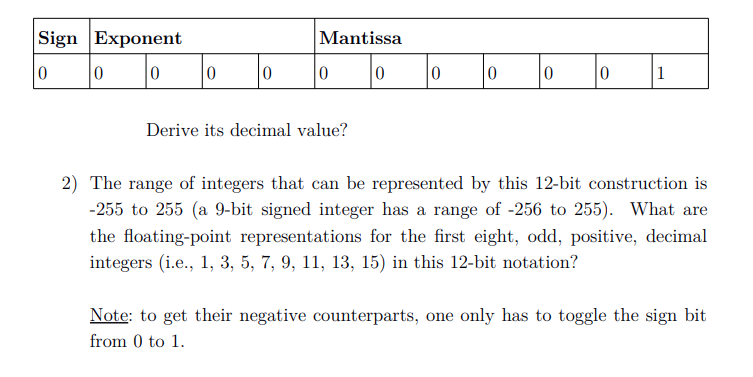# Sign ExponentMantissaDerive its decimal value?2) The range of integers that can be represented by this 12-bit construction is-255 to 255 (a 9-bit signed integer has a range of -256 to 255). What arethe floating-point representations for the first eight, odd, positive, decimalintegers (i.e., 1, 3, 5, 7, 9, 11, 13, 15) in this 12-bit notation?Note: to get their negative counterparts, one only has to toggle the sign bitfrom 0 to 1.

Question
6 views

What is the process of writing these odd numbers in this 12-bit floating point representationhelp_outlineImage TranscriptioncloseSign Exponent Mantissa Derive its decimal value? 2) The range of integers that can be represented by this 12-bit construction is -255 to 255 (a 9-bit signed integer has a range of -256 to 255). What are the floating-point representations for the first eight, odd, positive, decimal integers (i.e., 1, 3, 5, 7, 9, 11, 13, 15) in this 12-bit notation? Note: to get their negative counterparts, one only has to toggle the sign bit from 0 to 1. fullscreen
check_circle

Step 1

Approach-

If the number is positive, then the sign bit is 0, otherwise sign bit is 1.

Convert the number to binary form.

For obtaining the value of mantissa-

1.nnnn × 2y (in scientific notation)

Here, nnnn is the mantissa and y is the exponent. Mantissa is obtained by taking the bits except the first 1 in binary representation.

Since mantissa is represented in 7-bits, and mantissa is of 3 bits. So, fill the rest of bits by filing zero in it.

Calculate the exponent-

In floating point representation, the exponent obtained is added with a bias-

Bias = (2a / 2) – 1

a =no of bits in exponent

Now add bias value to the exponent value and represent that number in binary form.

Step 2

Solution-

1) 1

As, the number is positive so sign bit will be 0.

Binary representation of 1 = 0001

In scientific form it will be represented as 1 × 20

So, exponent is 0.

Since we have no bits after 1 so the mantissa part will be 0000000

Bias = (24/2)-1 = 7

Now add 7 as bias value to exponent value, we will get 7 as answer.

Now represent 7 in binary form, which will be 0111.

So, the exponent bits will be 0111.

Hence, the final representation is shown below-

Step 3

2)  3

As, the number is positive so sign bit will be 0.

Binary representation of 3 = 0011

In scientific form it will be represented as 1.1 × 21

So, exponent is 1.

Since we must take bits after first 1 so the mantissa part will be 1000000

Bias = (24/2)-1 = 7

Now add 7 as bias value to expo...

### Want to see the full answer?

See Solution

#### Want to see this answer and more?

Solutions are written by subject experts who are available 24/7. Questions are typically answered within 1 hour.*

See Solution
*Response times may vary by subject and question.
Tagged in

### Computer Science